Research papers and code for "Enoch Yeung":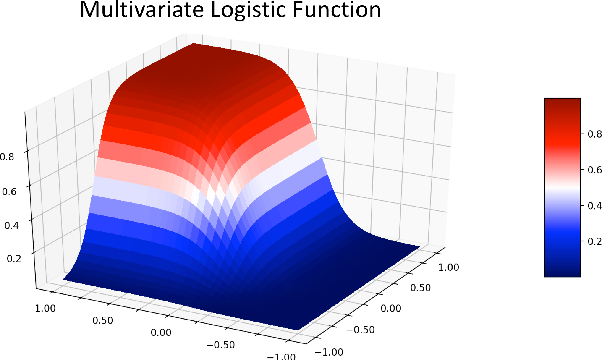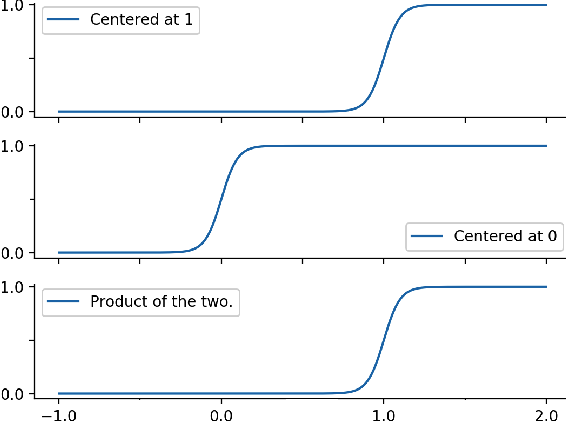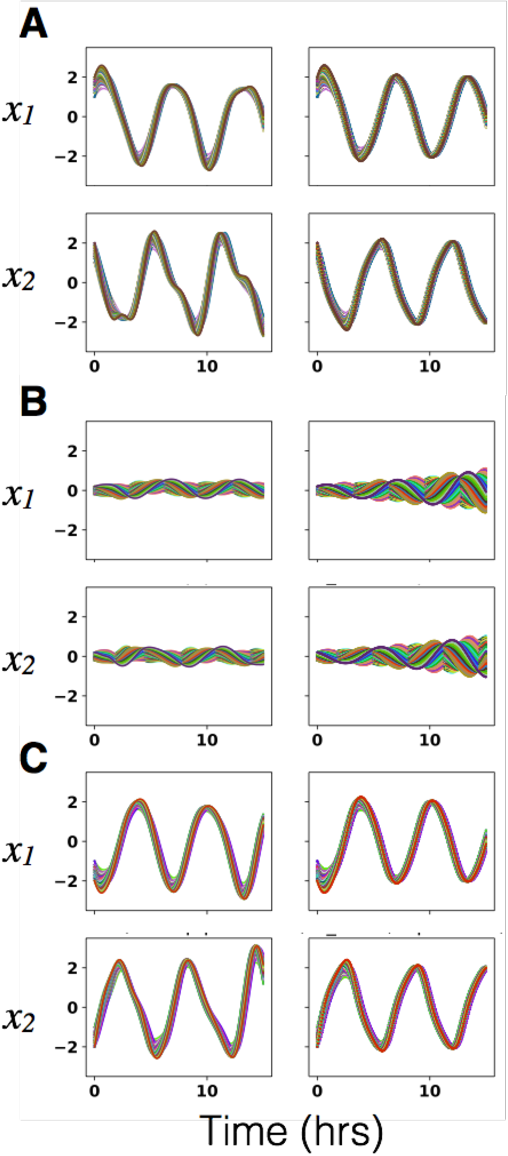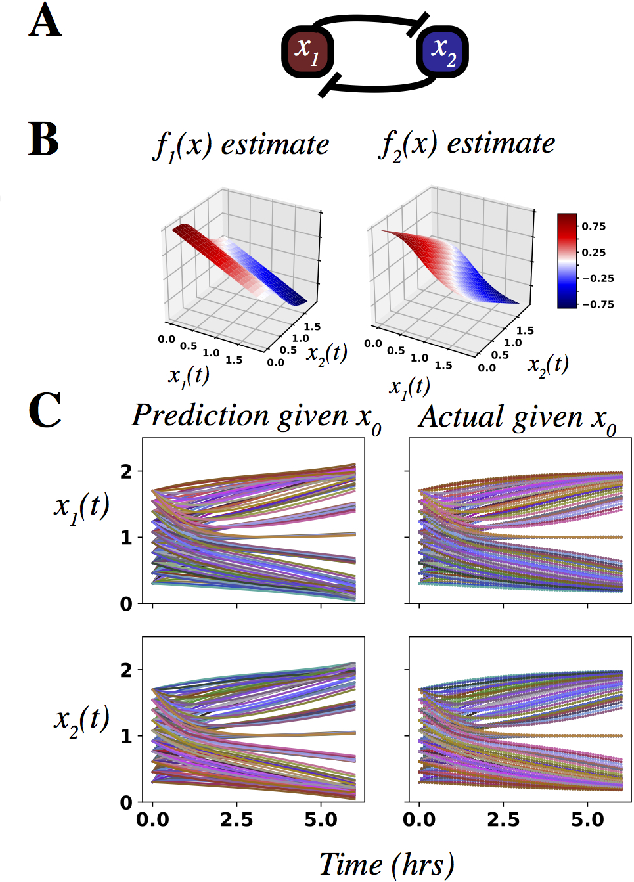An outstanding challenge in nonlinear systems theory is identification or learning of a given nonlinear system's Koopman operator directly from data or models. Advances in extended dynamic mode decomposition approaches and machine learning methods have enabled data-driven discovery of Koopman operators, for both continuous and discrete-time systems. Since Koopman operators are often infinite-dimensional, they are approximated in practice using finite-dimensional systems. The fidelity and convergence of a given finite-dimensional Koopman approximation is a subject of ongoing research. In this paper we introduce a class of Koopman observable functions that confer an approximate closure property on their corresponding finite-dimensional approximations of the Koopman operator. We derive error bounds for the fidelity of this class of observable functions, as well as identify two key learning parameters which can be used to tune performance. We illustrate our approach on two classical nonlinear system models: the Van Der Pol oscillator and the bistable toggle switch.

* 8 pages
Click to Read Paper and Get Code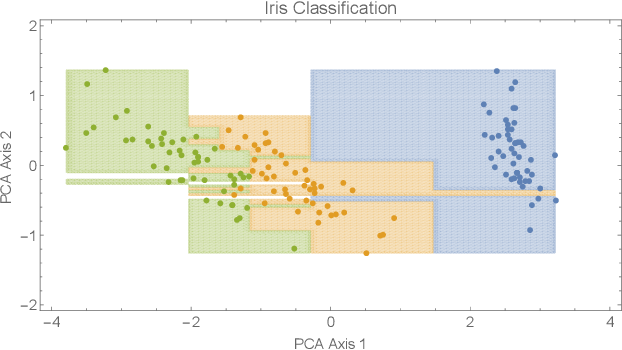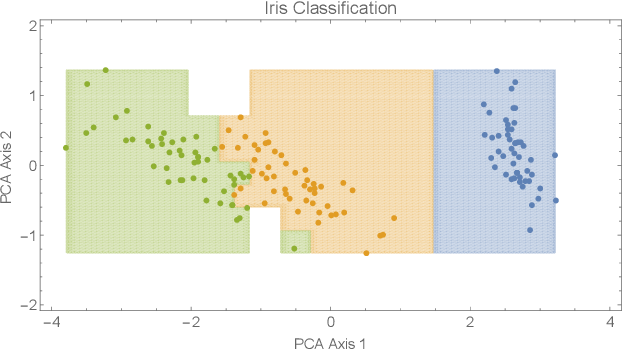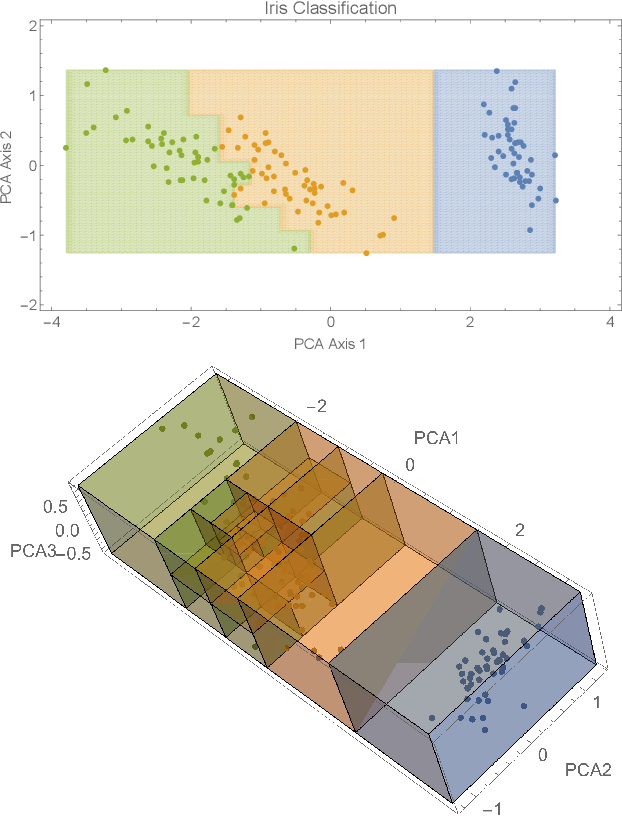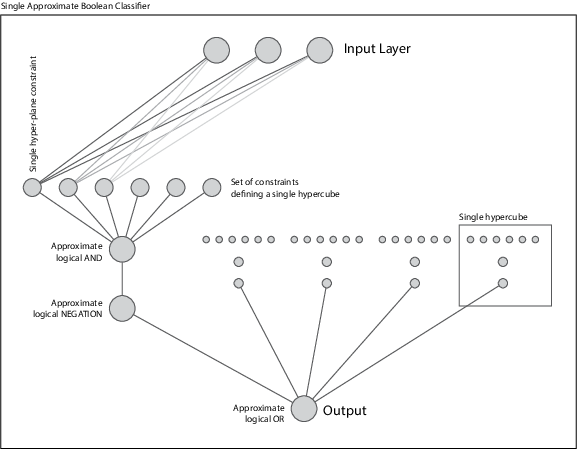In this paper we presented a novel constructive approach for training deep neural networks using geometric approaches. We show that a topological covering can be used to define a class of distributed linear matrix inequalities, which in turn directly specify the shape and depth of a neural network architecture. The key insight is a fundamental relationship between linear matrix inequalities and their ability to bound the shape of data, and the rectified linear unit (ReLU) activation function employed in modern neural networks. We show that unit cover geometry and cover porosity are two design variables in cover-constructive learning that play a critical role in defining the complexity of the model and generalizability of the resulting neural network classifier. In the context of cover-constructive learning, these findings underscore the age old trade-off between model complexity and overfitting (as quantified by the number of elements in the data cover) and generalizability on test data. Finally, we benchmark on algorithm on the Iris, MNIST, and Wine dataset and show that the constructive algorithm is able to train a deep neural network classifier in one shot, achieving equal or superior levels of training and test classification accuracy with reduced training time.

Click to Read Paper and Get Code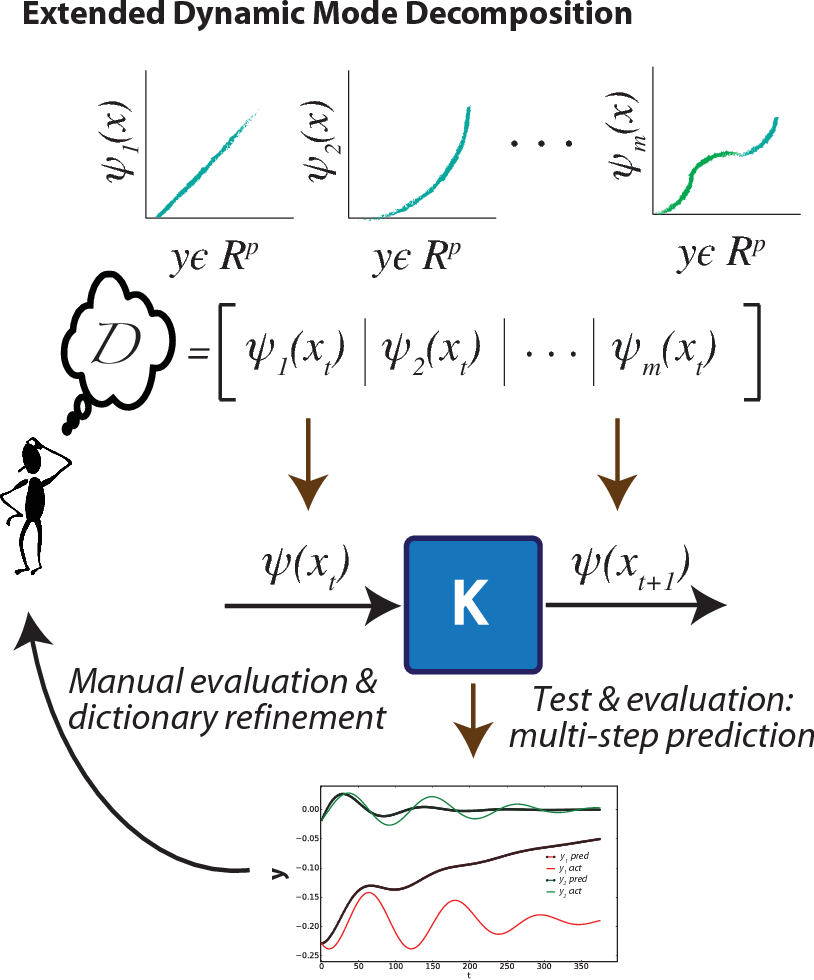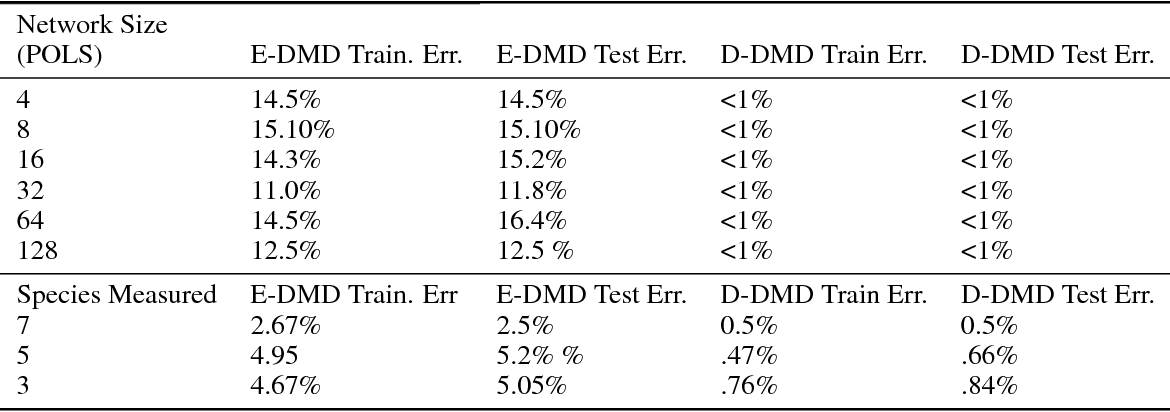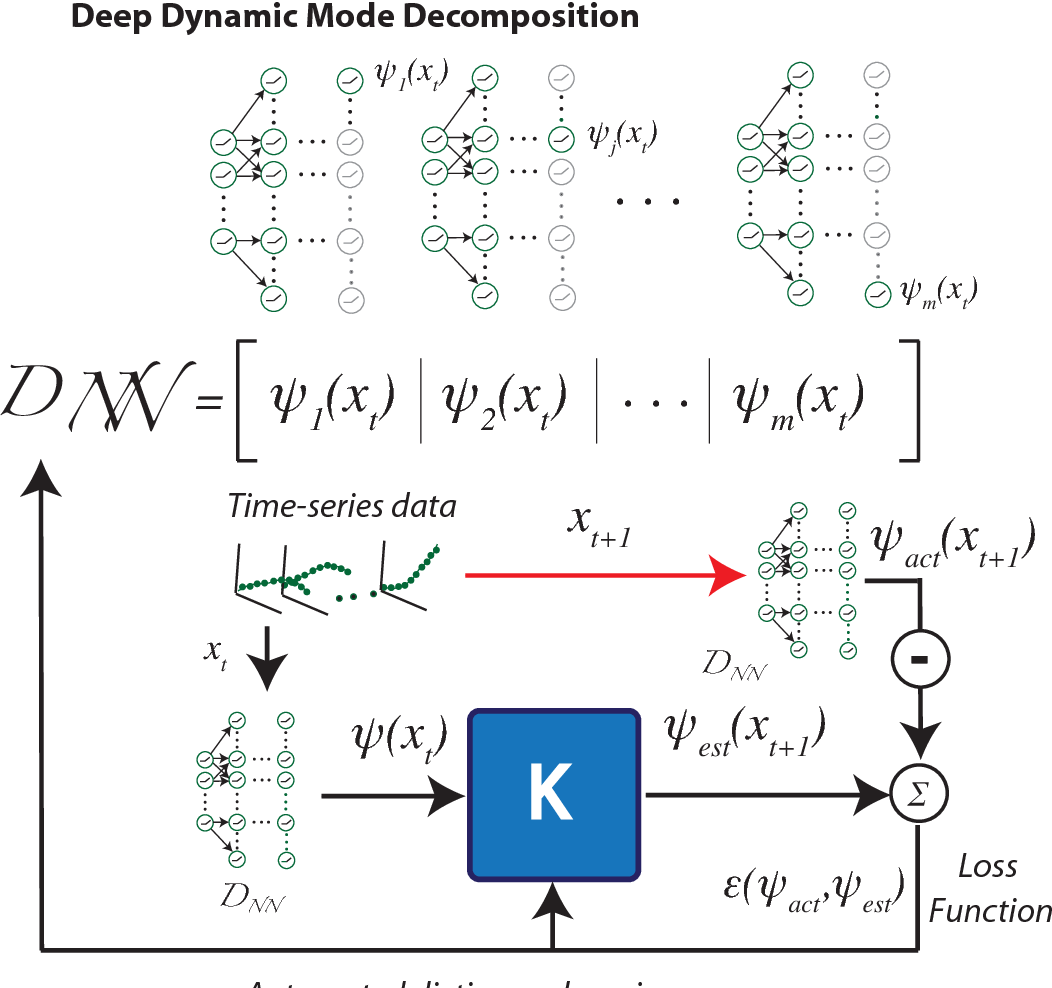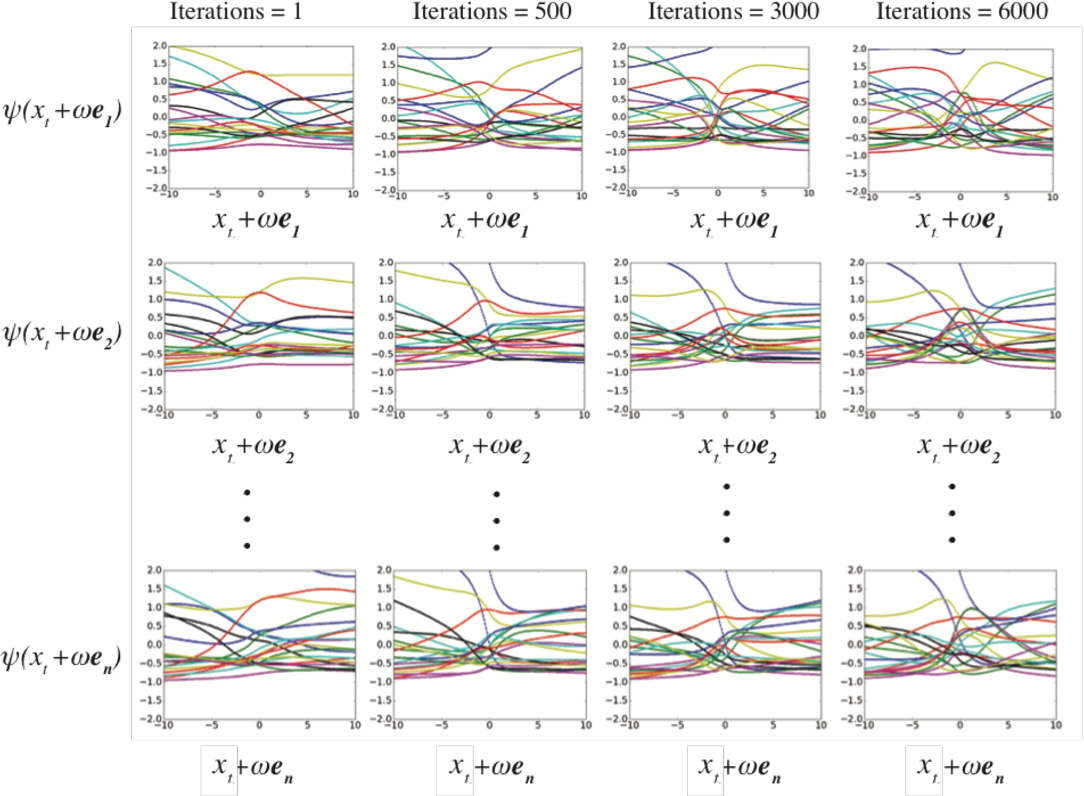The Koopman operator has recently garnered much attention for its value in dynamical systems analysis and data-driven model discovery. However, its application has been hindered by the computational complexity of extended dynamic mode decomposition; this requires a combinatorially large basis set to adequately describe many nonlinear systems of interest, e.g. cyber-physical infrastructure systems, biological networks, social systems, and fluid dynamics. Often the dictionaries generated for these problems are manually curated, requiring domain-specific knowledge and painstaking tuning. In this paper we introduce a deep learning framework for learning Koopman operators of nonlinear dynamical systems. We show that this novel method automatically selects efficient deep dictionaries, outperforming state-of-the-art methods. We benchmark this method on partially observed nonlinear systems, including the glycolytic oscillator and show it is able to predict quantitatively 100 steps into the future, using only a single timepoint, and qualitative oscillatory behavior 400 steps into the future.

* 16 pages, 5 figures
Click to Read Paper and Get Code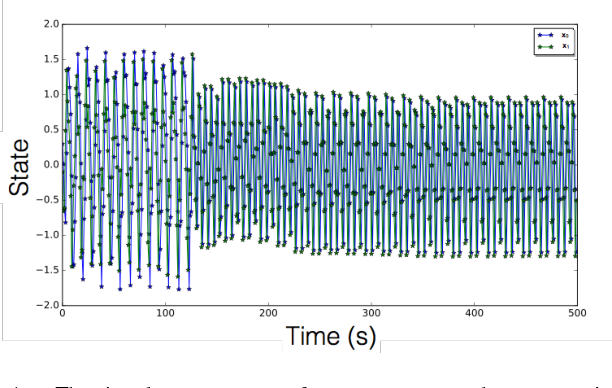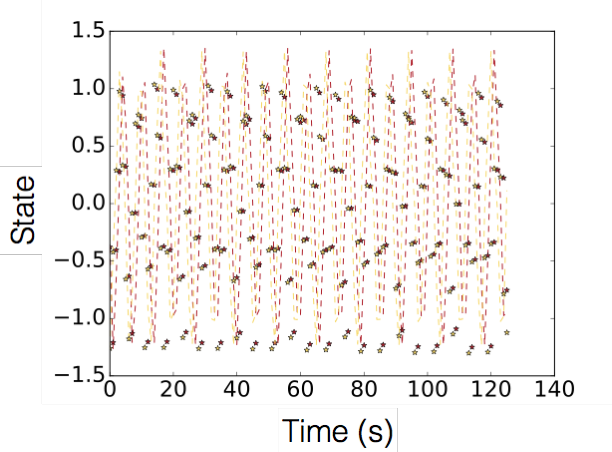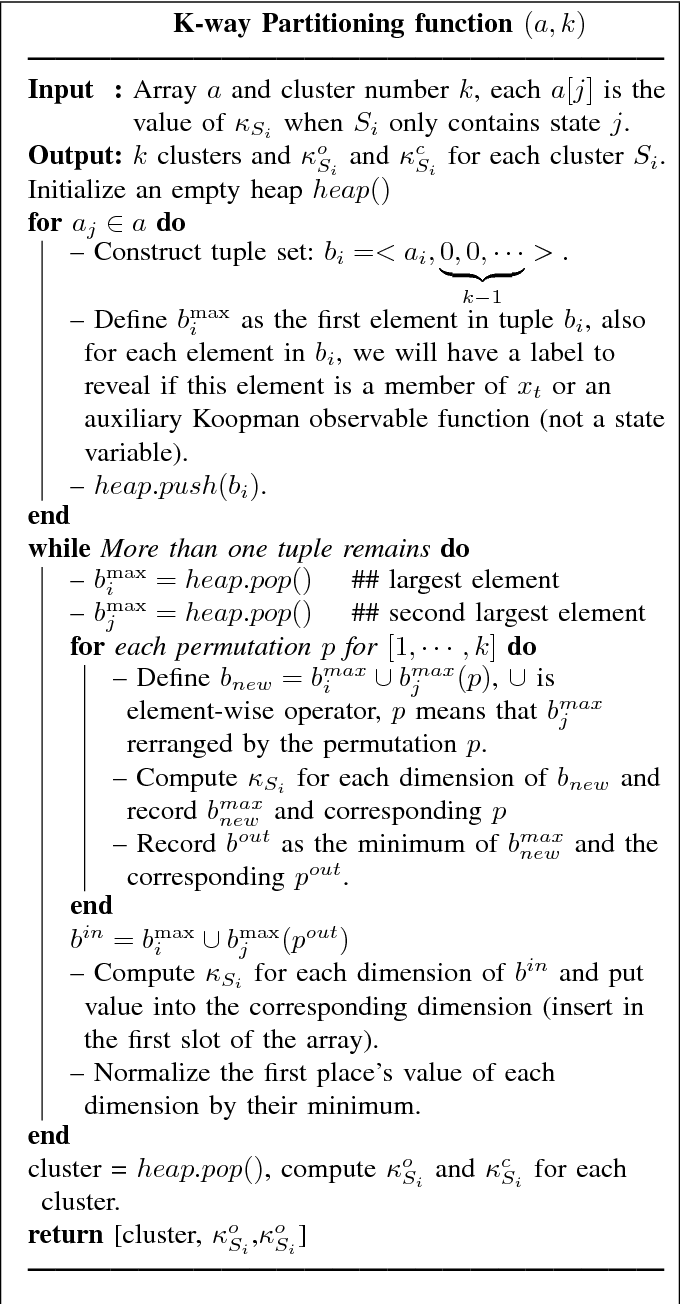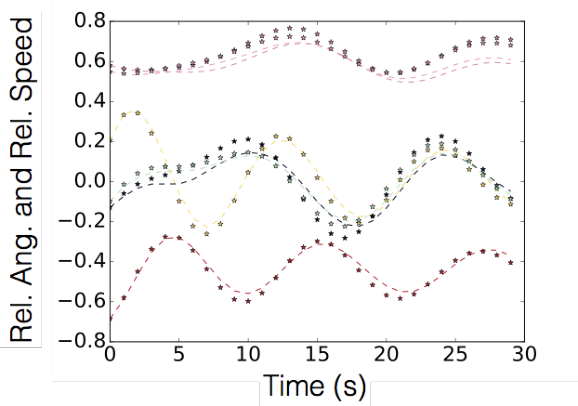In this paper we propose a new Koopman operator approach to the decomposition of nonlinear dynamical systems using Koopman Gramians. We introduce the notion of an input-Koopman operator, and show how input-Koopman operators can be used to cast a nonlinear system into the classical state-space form, and identify conditions under which input and state observable functions are well separated. We then extend an existing method of dynamic mode decomposition for learning Koopman operators from data known as deep dynamic mode decomposition to systems with controls or disturbances. We illustrate the accuracy of the method in learning an input-state separable Koopman operator for an example system, even when the underlying system exhibits mixed state-input terms. We next introduce a nonlinear decomposition algorithm, based on Koopman Gramians, that maximizes internal subsystem observability and disturbance rejection from unwanted noise from other subsystems. We derive a relaxation based on Koopman Gramians and multi-way partitioning for the resulting NP-hard decomposition problem. We lastly illustrate the proposed algorithm with the swing dynamics for an IEEE 39-bus system.

* 8 pages, submitted to IEEE 2018 ACC
Click to Read Paper and Get Code
Generative Adversarial Networks (GANs) are becoming popular choices for unsupervised learning. At the same time there is a concerted effort in the machine learning community to expand the range of tasks in which learning can be applied as well as to utilize methods from other disciplines to accelerate learning. With this in mind, in the current work we suggest ways to enforce given constraints in the output of a GAN both for interpolation and extrapolation. The two cases need to be treated differently. For the case of interpolation, the incorporation of constraints is built into the training of the GAN. The incorporation of the constraints respects the primary game-theoretic setup of a GAN so it can be combined with existing algorithms. However, it can exacerbate the problem of instability during training that is well-known for GANs. We suggest adding small noise to the constraints as a simple remedy that has performed well in our numerical experiments. The case of extrapolation (prediction) is more involved. First, we employ a modified interpolation training process that uses noisy data but does not necessarily enforce the constraints during training. Second, the resulting modified interpolator is used for extrapolation where the constraints are enforced after each step through projection on the space of constraints.

* 29 pages
Click to Read Paper and Get Code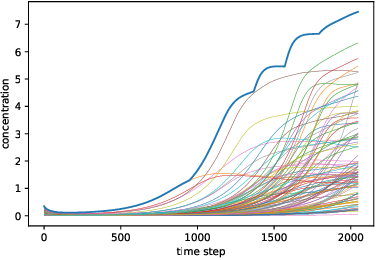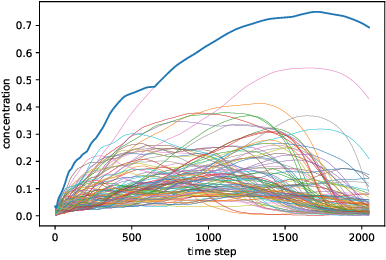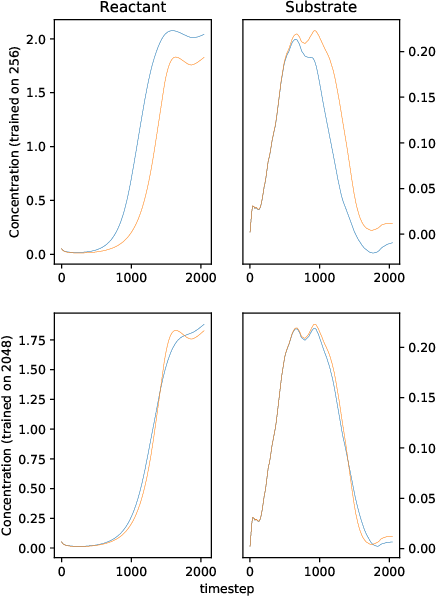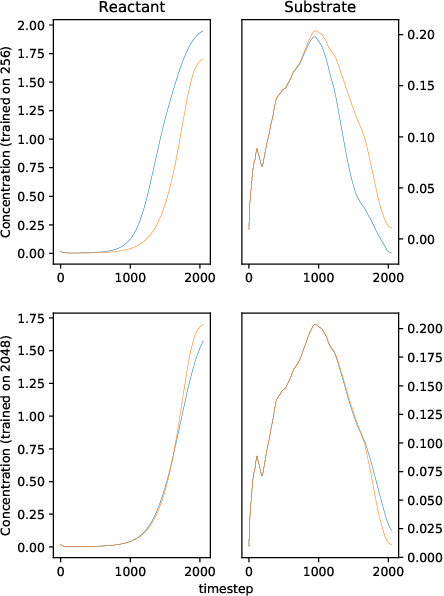We solve a system of ordinary differential equations with an unknown functional form of a sink (reaction rate) term. We assume that the measurements (time series) of state variables are partially available, and we use recurrent neural network to "learn" the reaction rate from this data. This is achieved by including a discretized ordinary differential equations as part of a recurrent neural network training problem. We extend TensorFlow's recurrent neural network architecture to create a simple but scalable and effective solver for the unknown functions, and apply it to a fedbatch bioreactor simulation problem. Use of techniques from recent deep learning literature enables training of functions with behavior manifesting over thousands of time steps. Our networks are structurally similar to recurrent neural networks, but differences in design and function require modifications to the conventional wisdom about training such networks.

* 19 pages, 8 figures
Click to Read Paper and Get Code